# ISEE Lower Level Math : How to find the part from the whole

## Example Questions

1 2 3 4 6 Next →

### Example Question #79 : Whole And Part

What isof?Explanation:

To find a fraction of a whole number, we will multiply the fraction by the whole number.  So, we get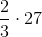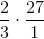We can simplify before we multiply.  The 3 and the 27 can both be divided by 3.  So, we get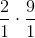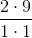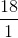Therefore,ofis.

### Example Question #80 : Whole And Part

What isof?Explanation:

To find a fraction of a whole number, we will multiply the two together.  So, we get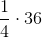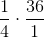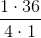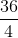1 2 3 4 6 Next →